1597235100

# k nearest neighbors computational complexity

### Understanding the computational cost of kNN algorithm, with case study examples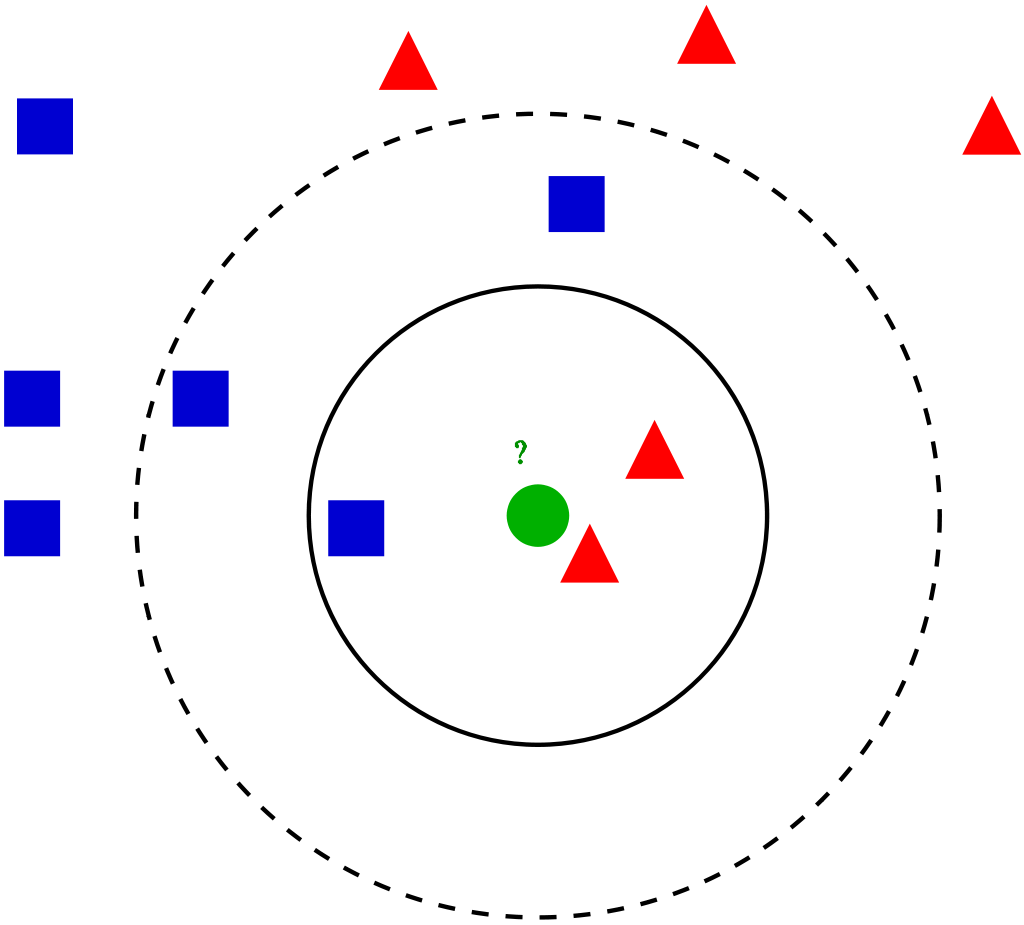Visualization of the kNN algorithm (source)

## Algorithm introduction

kNN (k nearest neighbors) is one of the simplest ML algorithms, often taught as one of the first algorithms during introductory courses. It’s relatively simple but quite powerful, although rarely time is spent on understanding its computational complexity and practical issues. It can be used both for classification and regression with the same complexity, so for simplicity, we’ll consider the kNN classifier.

kNN is an associative algorithm — during prediction it searches for the nearest neighbors and takes their majority vote as the class predicted for the sample. Training phase may or may not exist at all, as in general, we have 2 possibilities:

1. Brute force method — calculate distance from new point to every point in training data matrix X, sort distances and take k nearest, then do a majority vote. There is no need for separate training, so we only consider prediction complexity.
2. Using data structure — organize the training points from X into the auxiliary data structure for faster nearest neighbors lookup. This approach uses additional space and time (for creating data structure during training phase) for faster predictions.

We focus on the methods implemented in Scikit-learn, the most popular ML library for Python. It supports brute force, k-d tree and ball tree data structures. These are relatively simple, efficient and perfectly suited for the kNN algorithm. Construction of these trees stems from computational geometry, not from machine learning, and does not concern us that much, so I’ll cover it in less detail, more on the conceptual level. For more details on that, see links at the end of the article.

In all complexities below times of calculating the distance were omitted since they are in most cases negligible compared to the rest of the algorithm. Additionally, we mark:

• `n`: number of points in the training dataset
• `d`: data dimensionality
• `k`: number of neighbors that we consider for voting

## Brute force method

Training time complexity: `O(1)`

**Training space complexity: **`O(1)`

Prediction time complexity: `O(k * n)`

Prediction space complexity: `O(1)`

Training phase technically does not exist, since all computation is done during prediction, so we have `O(1)` for both time and space.

Prediction phase is, as method name suggest, a simple exhaustive search, which in pseudocode is:

``````Loop through all points k times:
1\. Compute the distance between currently classifier sample and
training points, remember the index of the element with the
smallest distance (ignore previously selected points)
2\. Add the class at found index to the counter
Return the class with the most votes as a prediction
``````

This is a nested loop structure, where the outer loop takes `k` steps and the inner loop takes `n` steps. 3rd point is `O(1)` and 4th is `O(## of classes)`, so they are smaller. Therefore, we have `O(n * k)` time complexity.

As for space complexity, we need a small vector to count the votes for each class. It’s almost always very small and is fixed, so we can treat is as a `O(1)` space complexity.

#k-nearest-neighbours #knn-algorithm #knn #machine-learning #algorithms

## Buddha Community1597235100

## k nearest neighbors computational complexity

### Understanding the computational cost of kNN algorithm, with case study examplesVisualization of the kNN algorithm (source)

## Algorithm introduction

kNN (k nearest neighbors) is one of the simplest ML algorithms, often taught as one of the first algorithms during introductory courses. It’s relatively simple but quite powerful, although rarely time is spent on understanding its computational complexity and practical issues. It can be used both for classification and regression with the same complexity, so for simplicity, we’ll consider the kNN classifier.

kNN is an associative algorithm — during prediction it searches for the nearest neighbors and takes their majority vote as the class predicted for the sample. Training phase may or may not exist at all, as in general, we have 2 possibilities:

1. Brute force method — calculate distance from new point to every point in training data matrix X, sort distances and take k nearest, then do a majority vote. There is no need for separate training, so we only consider prediction complexity.
2. Using data structure — organize the training points from X into the auxiliary data structure for faster nearest neighbors lookup. This approach uses additional space and time (for creating data structure during training phase) for faster predictions.

We focus on the methods implemented in Scikit-learn, the most popular ML library for Python. It supports brute force, k-d tree and ball tree data structures. These are relatively simple, efficient and perfectly suited for the kNN algorithm. Construction of these trees stems from computational geometry, not from machine learning, and does not concern us that much, so I’ll cover it in less detail, more on the conceptual level. For more details on that, see links at the end of the article.

In all complexities below times of calculating the distance were omitted since they are in most cases negligible compared to the rest of the algorithm. Additionally, we mark:

• `n`: number of points in the training dataset
• `d`: data dimensionality
• `k`: number of neighbors that we consider for voting

## Brute force method

Training time complexity: `O(1)`

**Training space complexity: **`O(1)`

Prediction time complexity: `O(k * n)`

Prediction space complexity: `O(1)`

Training phase technically does not exist, since all computation is done during prediction, so we have `O(1)` for both time and space.

Prediction phase is, as method name suggest, a simple exhaustive search, which in pseudocode is:

``````Loop through all points k times:
1\. Compute the distance between currently classifier sample and
training points, remember the index of the element with the
smallest distance (ignore previously selected points)
2\. Add the class at found index to the counter
Return the class with the most votes as a prediction
``````

This is a nested loop structure, where the outer loop takes `k` steps and the inner loop takes `n` steps. 3rd point is `O(1)` and 4th is `O(## of classes)`, so they are smaller. Therefore, we have `O(n * k)` time complexity.

As for space complexity, we need a small vector to count the votes for each class. It’s almost always very small and is fixed, so we can treat is as a `O(1)` space complexity.

#k-nearest-neighbours #knn-algorithm #knn #machine-learning #algorithms1641805837

## How to Predict Housing Prices with Linear Regression?The final objective is to estimate the cost of a certain house in a Boston suburb. In 1970, the Boston Standard Metropolitan Statistical Area provided the information. To examine and modify the data, we will use several techniques such as data pre-processing and feature engineering. After that, we'll apply a statistical model like regression model to anticipate and monitor the real estate market.

Project Outline:

• EDA
• Feature Engineering
• Pick and Train a Model
• Interpret
• Conclusion

### EDA

Before using a statistical model, the EDA is a good step to go through in order to:

• Recognize the data set
• Check to see if any information is missing.
• Find some outliers.
• To get more out of the data, add, alter, or eliminate some features.

### Importing the Libraries

• Recognize the data set
• Check to see if any information is missing.
• Find some outliers.
• To get more out of the data, add, alter, or eliminate some features.

# Import the libraries #Dataframe/Numerical libraries import pandas as pd import numpy as np #Data visualization import plotly.express as px import matplotlib import matplotlib.pyplot as plt import seaborn as sns #Machine learning model from sklearn.linear_model import LinearRegression

### Have a Look at the Columns

Crime: It refers to a town's per capita crime rate.

ZN: It is the percentage of residential land allocated for 25,000 square feet.

Indus: The amount of non-retail business lands per town is referred to as the indus.

CHAS: CHAS denotes whether or not the land is surrounded by a river.

NOX: The NOX stands for nitric oxide content (part per 10m)

RM: The average number of rooms per home is referred to as RM.

AGE: The percentage of owner-occupied housing built before 1940 is referred to as AGE.

DIS: Weighted distance to five Boston employment centers are referred to as dis.

TAX: The TAX columns denote the rate of full-value property taxes per \$10,000 dollars.

B: B=1000(Bk — 0.63)2 is the outcome of the equation, where Bk is the proportion of blacks in each town.

PTRATIO: It refers to the student-to-teacher ratio in each community.

LSTAT: It refers to the population's lower socioeconomic status.

MEDV: It refers to the 1000-dollar median value of owner-occupied residences.

### Data Preprocessing

# Check if there is any missing values. housing_df.isna().sum() CRIM       0 ZN         0 INDUS      0 CHAS       0 NOX        0 RM         0 AGE        0 DIS        0 RAD        0 TAX        0 PTRATIO    0 B          0 LSTAT      0 MEDV       0 dtype: int64

No missing values are found

We examine our data's mean, standard deviation, and percentiles.

housing_df.describe()The crime, area, sector, nitric oxides, 'B' appear to have multiple outliers at first look because the minimum and maximum values are so far apart. In the Age columns, the mean and the Q2(50 percentile) do not match.

We might double-check it by examining the distribution of each column.

### Inferences

1. The rate of crime is rather low. The majority of values are in the range of 0 to 25. With a huge value and a value of zero.
2. The majority of residential land is zoned for less than 25,000 square feet. Land zones larger than 25,000 square feet represent a small portion of the dataset.
3. The percentage of non-retial commercial acres is mostly split between two ranges: 0-13 and 13-23.
4. The majority of the properties are bordered by the river, although a tiny portion of the data is not.
5. The content of nitrite dioxide has been trending lower from.3 to.7, with a little bump towards.8. It is permissible to leave a value in the range of 0.1–1.
6. The number of rooms tends to cluster around the average.
7. With time, the proportion of owner-occupied units rises.
8. As the number of weights grows, the weight distance between 5 employment centers reduces. It could indicate that individuals choose to live in new high-employment areas.
9. People choose to live in places with limited access to roadways (0-10). We have a 30th percentile outlier.
10. The majority of dwelling taxes are in the range of \$200-450, with large outliers around \$700,000.
11. The percentage of people with lower status tends to cluster around the median. The majority of persons are of lower social standing.

Because the model is overly generic, removing all outliers will underfit it. Keeping all outliers causes the model to overfit and become excessively accurate. The data's noise will be learned.

The approach is to establish a happy medium that prevents the model from becoming overly precise. When faced with a new set of data, however, they generalise well.

We'll keep numbers below 600 because there's a huge anomaly in the TAX column around 600.

new_df=housing_df[housing_df['TAX']<600]

### Looking at the DistributionThe overall distribution, particularly the TAX, PTRATIO, and RAD, has improved slightly.

### CorrelationPerfect correlation is denoted by the clear values. The medium correlation between the columns is represented by the reds, while the negative correlation is represented by the black.

With a value of 0.89, we can see that 'MEDV', which is the medium price we wish to anticipate, is substantially connected with the number of rooms 'RM'. The proportion of black people in area 'B' with a value of 0.19 is followed by the residential land 'ZN' with a value of 0.32 and the percentage of black people in area 'ZN' with a value of 0.32.

The metrics that are most connected with price will be plotted.## Feature Engineering

### Feature Scaling

Gradient descent is aided by feature scaling, which ensures that all features are on the same scale. It makes locating the local optimum much easier.

Mean standardization is one strategy to employ. It substitutes (target-mean) for the target to ensure that the feature has a mean of nearly zero.

def standard(X):    '''Standard makes the feature 'X' have a zero mean'''    mu=np.mean(X) #mean    std=np.std(X) #standard deviation    sta=(X-mu)/std # mean normalization    return mu,std,sta     mu,std,sta=standard(X) X=sta X

### Choose and Train the Model

For the sake of the project, we'll apply linear regression.

Typically, we run numerous models and select the best one based on a particular criterion.

Linear regression is a sort of supervised learning model in which the response is continuous, as it relates to machine learning.

Form of Linear Regression

y= θX+θ1 or y= θ1+X1θ2 +X2θ3 + X3θ4

y is the target you will be predicting

0 is the coefficient

x is the input

We will Sklearn to develop and train the model

#Import the libraries to train the model from sklearn.model_selection import train_test_split from sklearn.linear_model import LinearRegression

Allow us to utilise the train/test method to learn a part of the data on one set and predict using another set using the train/test approach.

X_train,X_test,y_train,y_test=train_test_split(X,y,test_size=0.4) #Create and Train the model model=LinearRegression().fit(X_train,y_train) #Generate prediction predictions_test=model.predict(X_test) #Compute loss to evaluate the model coefficient= model.coef_ intercept=model.intercept_ print(coefficient,intercept) [7.22218258] 24.66379606613584

In this example, you will learn the model using below hypothesis:

Price= 24.85 + 7.18* Room

It is interpreted as:

For a decided price of a house:

A 7.18-unit increase in the price is connected with a growth in the number of rooms.

As a side note, this is an association, not a cause!

### Interpretation

You will need a metric to determine whether our hypothesis was right. The RMSE approach will be used.

Root Means Square Error (RMSE) is defined as the square root of the mean of square error. The difference between the true and anticipated numbers called the error. It's popular because it can be expressed in y-units, which is the median price of a home in our scenario.

def rmse(predict,actual):    return np.sqrt(np.mean(np.square(predict - actual))) # Split the Data into train and test set X_train,X_test,y_train,y_test=train_test_split(X,y,test_size=0.4) #Create and Train the model model=LinearRegression().fit(X_train,y_train) #Generate prediction predictions_test=model.predict(X_test) #Compute loss to evaluate the model coefficient= model.coef_ intercept=model.intercept_ print(coefficient,intercept) loss=rmse(predictions_test,y_test) print('loss: ',loss) print(model.score(X_test,y_test)) #accuracy [7.43327725] 24.912055881970886 loss: 3.9673165450580714 0.7552661033654667 Loss will be 3.96

This means that y-units refer to the median value of occupied homes with 1000 dollars.

This will be less by 3960 dollars.

While learning the model you will have a high variance when you divide the data. Coefficient and intercept will vary. It's because when we utilized the train/test approach, we choose a set of data at random to place in either the train or test set. As a result, our theory will change each time the dataset is divided.

This problem can be solved using a technique called cross-validation.

### Improvisation in the Model

With 'Forward Selection,' we'll iterate through each parameter to assist us choose the numbers characteristics to include in our model.

### Forward Selection

1. Choose the most appropriate variable (in our case based on high correlation)
2. Add the next best variable to the model
3. Some predetermined conditions must meet.

We'll use a random state of 1 so that each iteration yields the same outcome.

cols=[] los=[] los_train=[] scor=[] i=0 while i < len(high_corr_var):    cols.append(high_corr_var[i])        # Select inputs variables    X=new_df[cols]        #mean normalization    mu,std,sta=standard(X)    X=sta        # Split the data into training and testing    X_train,X_test,y_train,y_test= train_test_split(X,y,random_state=1)        #fit the model to the training    lnreg=LinearRegression().fit(X_train,y_train)        #make prediction on the training test    prediction_train=lnreg.predict(X_train)        #make prediction on the testing test    prediction=lnreg.predict(X_test)        #compute the loss on train test    loss=rmse(prediction,y_test)    loss_train=rmse(prediction_train,y_train)    los_train.append(loss_train)    los.append(loss)        #compute the score    score=lnreg.score(X_test,y_test)    scor.append(score)        i+=1

We have a big 'loss' with a smaller collection of variables, yet our system will overgeneralize in this scenario. Although we have a reduced 'loss,' we have a large number of variables. However, if the model grows too precise, it may not generalize well to new data.

In order for our model to generalize well with another set of data, we might use 6 or 7 features. The characteristic chosen is descending based on how strong the price correlation is.

high_corr_var ['RM', 'ZN', 'B', 'CHAS', 'RAD', 'DIS', 'CRIM', 'NOX', 'AGE', 'TAX', 'INDUS', 'PTRATIO', 'LSTAT']

With 'RM' having a high price correlation and LSTAT having a negative price correlation.

# Create a list of features names feature_cols=['RM','ZN','B','CHAS','RAD','CRIM','DIS','NOX'] #Select inputs variables X=new_df[feature_cols] # Split the data into training and testing sets X_train,X_test,y_train,y_test= train_test_split(X,y, random_state=1) # feature engineering mu,std,sta=standard(X) X=sta # fit the model to the trainning data lnreg=LinearRegression().fit(X_train,y_train) # make prediction on the testing test prediction=lnreg.predict(X_test) # compute the loss loss=rmse(prediction,y_test) print('loss: ',loss) lnreg.score(X_test,y_test) loss: 3.212659865936143 0.8582338376696363

The test set yielded a loss of 3.21 and an accuracy of 85%.

Other factors, such as alpha, the learning rate at which our model learns, could still be tweaked to improve our model. Alternatively, return to the preprocessing section and working to increase the parameter distribution.

For more details regarding scraping real estate data you can contact Scraping Intelligence today

https://www.websitescraper.com/how-to-predict-housing-prices-with-linear-regression.php1596905700

## K-Nearest Neighbors Algorithm

KNN is a non-parametric and lazy learning algorithm. Non-parametric means there is no assumption for underlying data distribution. In other words, the model structure determined from the dataset. This will be very helpful in practice where most of the real-world datasets do not follow mathematical theoretical assumptions.

KNN is one of the most simple and traditional non-parametric techniques to classify samples. Given an input vector, KNN calculates the approximate distances between the vectors and then assign the points which are not yet labeled to the class of its K-nearest neighbors.

The lazy algorithm means it does not need any training data points for model generation. All training data used in the testing phase. This makes training faster and the testing phase slower and costlier. The costly testing phase means time and memory. In the worst case, KNN needs more time to scan all data points, and scanning all data points will require more memory for storing training data.

## K-NN for classification

Classification is a type of supervised learning. It specifies the class to which data elements belong to and is best used when the output has finite and discrete values. It predicts a class for an input variable as well.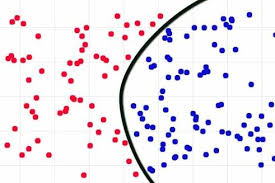Consider given review is positive (or) Negative, classification is all about if we give a new query points determine (or) predict the given review is positive (or) Negative.

Classification is all about learning the function for given points.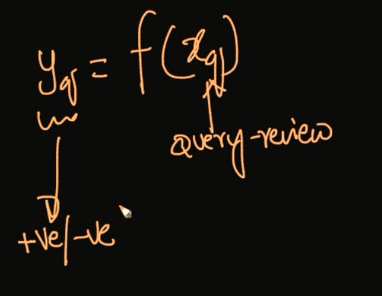How does the K-NN algorithm work?

In K-NN, K is the number of nearest neighbors. The number of neighbors is the core deciding factor. K is generally an odd number if the number of classes is 2. When K=1, then the algorithm is known as the nearest neighbor algorithm. This is the simplest case. Suppose P1 is the point, for which label needs to predict. First, you find the one closest point to P1 and then the label of the nearest point assigned to P1.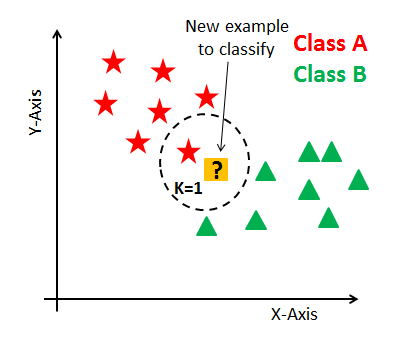Suppose P1 is the point, for which label needs to predict. First, you find the k closest point to P1 and then classify points by majority vote of its k neighbors. Each object votes for their class and the class with the most votes is taken as the prediction. For finding closest similar points, you find the distance between points using distance measures such as Euclidean distance, Hamming distance, Manhattan distance, and Minkowski distance.

K-NN has the following basic steps:

1. Calculate distance
2. Find closest neighbors
3. Vote for labels
4. Take the majority Vote

Failure Cases of K-NN:

_1.When Query Point is far away from the data points.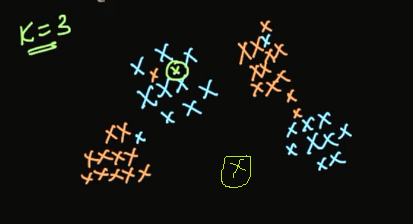2.If we have Jumble data sets.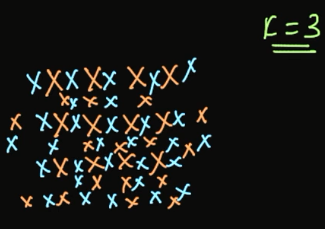For the above image shows jumble sets of data set, no useful information in the above data set. In this situation, the algorithm may be failing.

_Distance Measures in K-NN: _There are mainly four distance measures in Machine Learning Listed below.

1. Euclidean Distance
2. Manhattan Distance
3. Minkowski Distance
4. Hamming Distance

Euclidean Distance

The Euclidean distance between two points in either the plane or 3-dimensional space measures the length of a segment connecting the two points. It is the most obvious way of representing distance between two points. Euclidean distance marks the shortest route of the two points.

The Pythagorean Theorem can be used to calculate the distance between two points, as shown in the figure below. If the points (x1,y1)(x1,y1) and (x2,y2)(x2,y2) are in 2-dimensional space, then the Euclidean distance between them is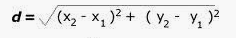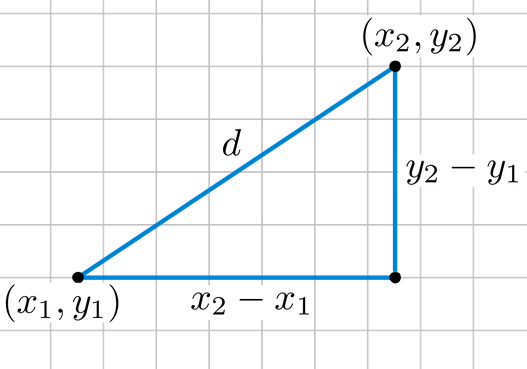Euclidean distance is called an L2 Norm of a vector.

Norm means the distance between two vectors.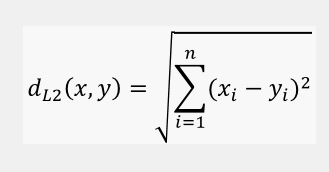Euclidean distance from an origin is given by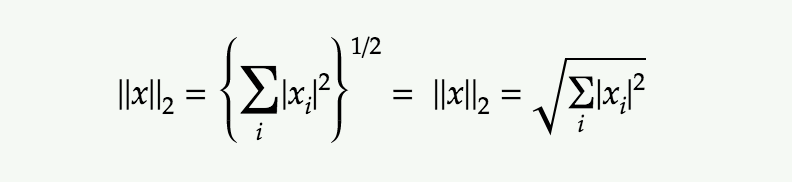Manhattan Distance

The Manhattan distance between two vectors (city blocks) is equal to the one-norm of the distance between the vectors. The distance function (also called a “metric”) involved is also called the “taxi cab” metric.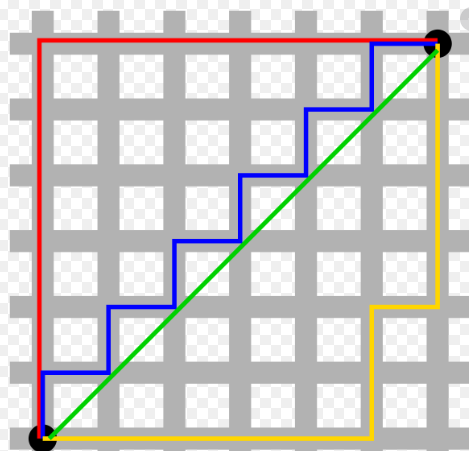Manhattan distance between two vectors is called as L1 Norm of a vector.

In L2 Norm we take the sum of the Squaring of the difference between elements vectors, in L1 Norm we take the sum of the absolute difference between elements vectors.

Manhattan Distance between two points (x1, y1) and (x2, y2) is:

|x1 — x2| + |y1 — y2|.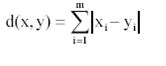Manhattan Distance****from an origin is given by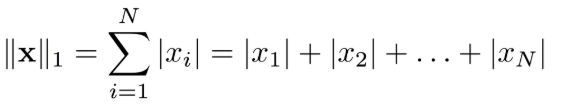Minkowski Distance

Minkowski distance__is a metric in a normed vector space. Minkowski distance is used for distance similarity of vector. Given two or more vectors, find distance similarity of these vectors.

#analytics #machine-learning #applied-ai #data-science #k-nearest-neighbors #data analytic1602745200

## Exploring The Brute Force K-Nearest Neighbors Algorithm

Did you find any difference between the two graphs?

Both show the accuracy of a classification problem for K values between 1 to 10.

Both of the graphs use the KNN classifier model with ‘Brute-force’ algorithm and ‘Euclidean’ distance metric on same dataset. Then why is there a difference in the accuracy between the two graphs?

Before answering that question, let me just walk you through the KNN algorithm pseudo code.

I hope all are familiar with k-nearest neighbour algorithm. If not, you can read the basics about it at https://www.analyticsvidhya.com/blog/2018/03/introduction-k-neighbours-algorithm-clustering/.

We can implement a KNN model by following the below steps:

2. Initialise the value of k
3. For getting the predicted class, iterate from 1 to total number of training data points
4. Calculate the distance between test data and each row of training data. Here we will use Euclidean distance as our distance metric since it’s the most popular method. Some of the other metrics that can be used are Chebyshev, cosine, etc.
5. Sort the calculated distances in ascending order based on distance values
6. Get top k rows from the sorted array
7. Get the most frequent class of these rows
8. Return the predicted class

#2020 oct tutorials # overviews #algorithms #k-nearest neighbors #machine learning #python1599448320

## Under the Hood of K-Nearest Neighbors (KNN) and Popular Model Validation Techniques

This article contains in-depth algorithm overviews of the K-Nearest Neighbors algorithm (Classification and Regression) as well as the following Model Validation techniques: Traditional Train/Test Split and Repeated K-Fold Cross Validation. The algorithm overviews include detailed descriptions of the methodologies and mathematics that occur internally with accompanying concrete examples. Also included are custom, fully functional/flexible frameworks of the above algorithms built from scratch using primarily NumPy. Finally, there is a fully integrated Case Study which deploys several of the custom frameworks (KNN-Classification, Repeated K-Fold Cross Validation) through a full Machine Learning workflow alongside the Iris Flowers dataset to find the optimal KNN model.

Please use the imports below to run any included code within your own notebook or coding environment.

``````import pandas as pd
import numpy as np
import matplotlib.pyplot as plt
import seaborn as sns
from statistics import mean,stdev
from itertools import combinations
import math
%matplotlib inline
``````

### KNN Algorithm Overview

K-Nearest Neighbors is a popular pattern recognition algorithm used in Supervised Machine Learning to handle both classification and regression-based tasks. At a high level, this algorithm operates according to an intuitive methodology:

New points target values are predicted according to the target values of the K most similar points stored in the model’s training data.

Traditionally, ‘similar’ is interpreted as some form of a distance calculation. Therefore, another way to interpret the KNN prediction methodology is that predictions are based off the K closest points within the training data, hence the name K-Nearest Neighbors. With the concept of distance introduced, a good initial question to answer is how distance will be computed. While there are several different mathematical metrics that are viewed as a form of computing distance, this study will highlight the 3 following distance metrics: Euclidean, Manhattan, and Chebyshev.

### KNN Algorithm Overview — Distance Metrics

Euclidean distance, based in the Pythagorean Theorem, finds the straight line distance between two points in space. In other words, this is equivalent to finding the shortest distance between two points by drawing a single line between Point A and Point B. Manhattan distance, based in taxicab geometry, is the sum of all N distances between Point A and Point B in N dimensional feature space. For example, in 2D space the Manhattan distance between Point A and Point B would be the sum of the vertical and horizontal distance. Chebyshev distance is the maximum distance between Point A and Point B in N dimensional feature space. For example, in 2D space the Chebyshev distance between Point A and Point B would be max(horizontal distance, vertical distance), in other words whichever distance is greater between the two distances.

Consider Point A = (A_1, A_2, … , A_N) and Point B = (B_1, B_2, … , B_N) both exist in N dimensional feature space. The distance between these two points can be described by the following formulas: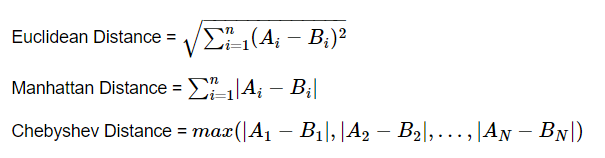As with most mathematical concepts, distance metrics are often easier to understand with a concrete example to visualize. Consider Point A = (0,0) and Point B = (3,4) in 2D feature space.

``````sns.set_style('darkgrid')
fig = plt.figure()
axes.scatter(x=[0,3],y=[0,4],s = 50)
axes.plot([0,3],[0,4],c='blue')
axes.annotate('X',[1.5,1.8],fontsize = 14,fontweight = 'bold')
axes.plot([0,0],[0,4],c='blue')
axes.annotate('Y',[-0.1,1.8],fontsize = 14,fontweight = 'bold')
axes.plot([0,3],[4,4],c='blue')
axes.annotate('Z',[1.5,4.1],fontsize = 14,fontweight = 'bold')
axes.annotate('(0,0)',[0.1,0.0],fontsize = 14,fontweight = 'bold')
axes.annotate('(3,4)',[2.85,3.7],fontsize = 14,fontweight = 'bold')
axes.grid(lw=1)
plt.show()
``````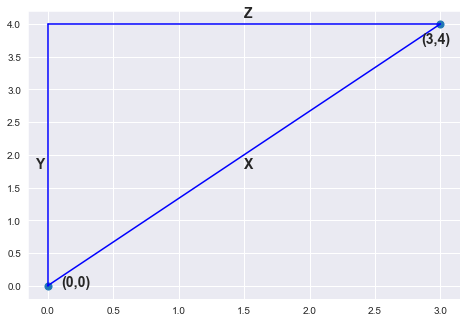#python #machine-learning #siri #k-nearest-neighbors #crossvalidation